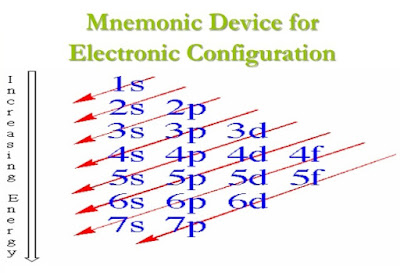Energy Levels, Sublevels and Atomic orbitalsBased from the flame test, Neils Bohr compared the model of an atom with that of the solar system. The nucleus of an atom is comparable to the sun the center of the atom and the Energy Levels are comparable to the orbits and the electrons are comparable to the planets.   Energy levels of an atom is where the electrons occupy.  Based from the Periodic Table of elements the biggest atom has 7 energy levels.  This principal energy levels of the atom has corresponding energy based from Bohr Theory, and and also composed of different sublevels and with different kind of orbitals.  As the energy level increases the energy required also increases.

Below is a table showing the different principal energy levels with their corresponding sublevels, orbitals and number of electrons:As you will notice the number of sublevels are just the same as the number of energy levels, and that the name of the sublevels are also the same as the name of orbitals.  In determining the number of orbitals n2 is used, where n represents the energy level and the maximum number of electrons can be determined using 2n2, where n is also the number of energy levels.

The sublevels are 1s, 2s, 2p, 3s, 3p, 3d, 4s, 4p, 4d, 5s, 5p, 5d, 5f, and 5g.  The last sublevel the 5g is not yet existing in the modern periodic table  There are also sublevels in 6th and 7th energy levels which will be shown below. These sublevels are not yet arranged according to their energies, the electrons distribution mnemonics found in the periodic table arranged the different sublevels according to increasing energy.The above electron distribution mnemonics can be used to write electron configuration and is already arranged according to increasing energy.  There is an overlapping in some sublevels, example 4s come first before 3d.  This is the arrangement following the above Mnemonics:

1s 2s 2p 3s 3p 4s 3d 4p 5s 4d 5p 6s 4f 5d 6p 7s 5f 6d 7p

This post first appeared on Science Concepts And Questions (K To 12), please read the originial post: here

Subscribe to Science Concepts And Questions (k To 12)

Get updates delivered right to your inbox!

×